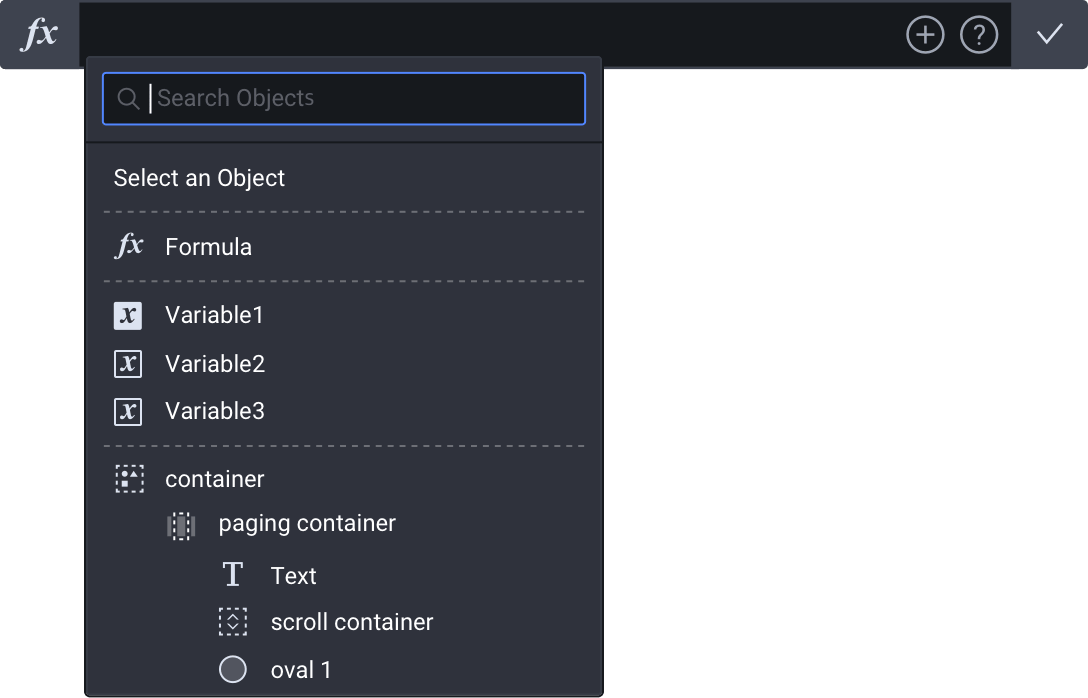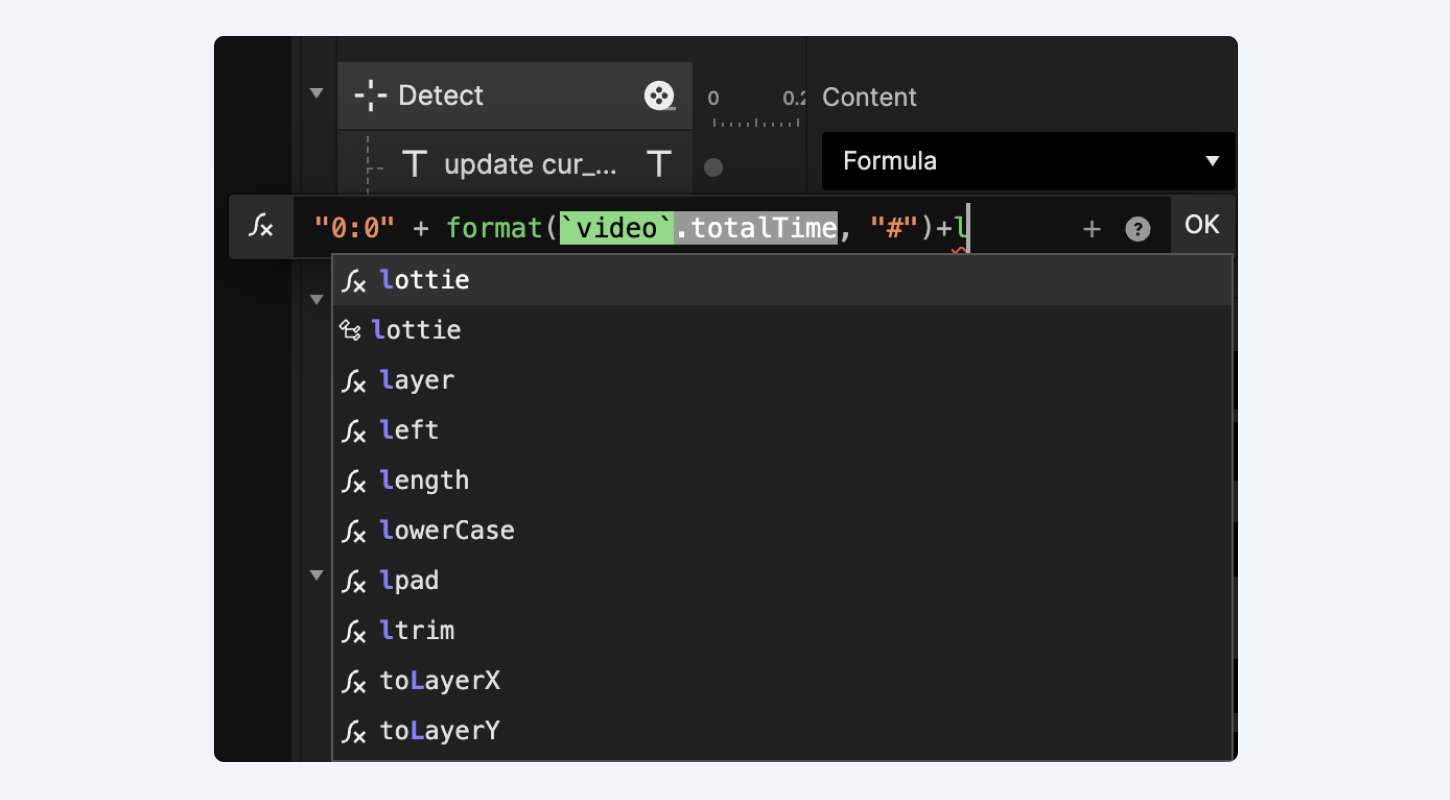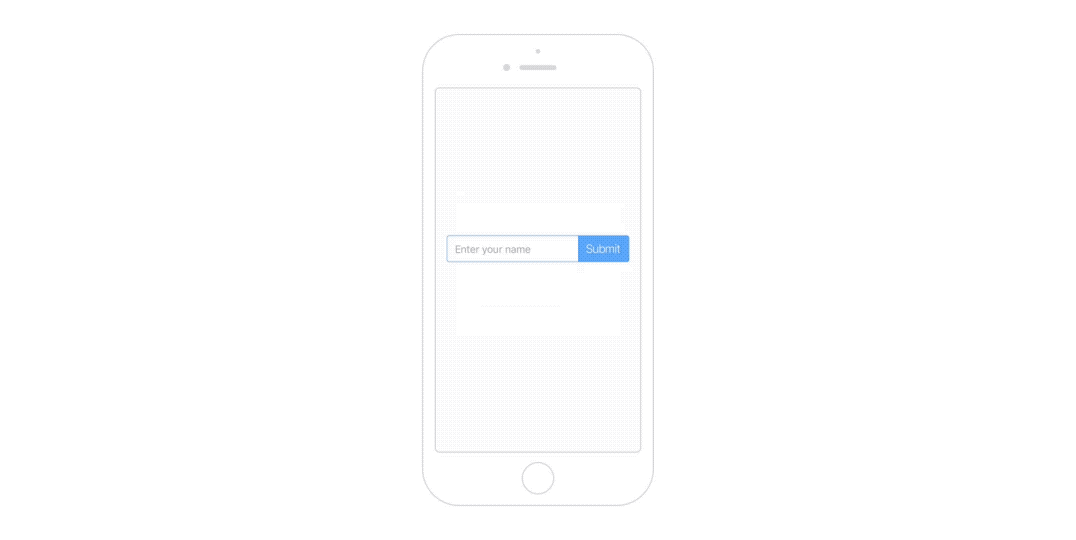# Formulas

With formulas and variables, it's possible to make prototypes with true dynamic interactions. A formula is an expression that "returns" a result. Use formulas to create dynamic interactions, e.g., password validation, counting the number of items in a shopping cart, and determining the total account balance.

Access layer properties, do calculations, and use the many functions to do, among others, text manipulation and advanced mathematical tasks.

The formula input field is where you create formulas. Include layer properties, functions, and variables in a formula as you wish. Click on the + icon to see the available layers and variables you can use in a formula.Enabling the Improved Formula Input Field in Labs allows you to identify whether a variable used inside a formula is local (can be used in the current scene only) or global (can be used in all scenes).

This distinction will help you visualize which function has been used in the formula, making it easier to check the formula's makeup. This can be especially useful when the pie was created by someone else.Completely new to formulas? Jumpstart with the examples below and the other sections to get the most out of formulas.

• Syntax — Any language has rules and principles to form sentences. For formulas, this isn't any different. Learn the syntax to create your first formula.
• Layer properties — There are plenty of layer properties to access. Using these is usually the first step to making dynamic interactions.
• Functions — Predefined formulas that perform a specific task. Functions usually "take in" data, process it, and "return" a result.
• Use cases — Practical use cases on formulas to learn from.

## Difference Between Formulas & Variables

First and foremost, formulas and variables do not exclude each other as you can use them independently from another. But, they do complement each other.

Think of a variable as a "basket" that stores a value. As a formula is an expression that "returns" a result, you might want to store this result to be used later. That's where variables come into play. To use the value stored in a variable, you need formulas.

To take it a step further, a variable could store an entire formula (instead of just the result). You would do this if you want to make a formula reusable across a scene.

Long story short, a formula could contain variables while a variable could contain a formula as well.

## Learning the Basics

Formulas don't have to be daunting. By adding a few formulas, you'll see that anyone can make dynamic interactions.

In this video, learn how to make an interaction involving a rectangle layer moving to where another rectangle layer is—anywhere in the scene.

## Practicing with Examples

### Making a Tab Bar

To make responses work dynamically, use formulas instead of fixed values. This example shows you how to use the x-coordinate of each menu layer for the Move responses. The blue layer will move left and right depending on whatever menu you tap on.### Using the Text from the Input Layer

To make a text layer display the text that was entered via a separate input layer, use the text property in a formula. In this example, the text property of the input layer refers to the entered text.